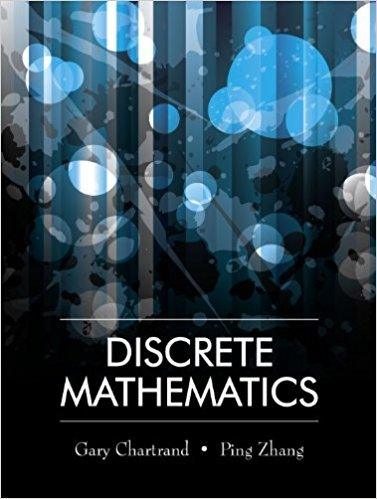×
×

# State the negation of each of the following statements. (a) 3 > 1. (b) The integer 0 isISBN: 9781577667308 385

## Solution for problem 2 Chapter 1.2

Discrete Mathematics | 1st Edition

• Textbook Solutions
• 2901 Step-by-step solutions solved by professors and subject experts
• Get 24/7 help from StudySoup virtual teaching assistantsDiscrete Mathematics | 1st Edition

4 5 1 337 Reviews
11
0
Problem 2

State the negation of each of the following statements. (a) 3 > 1. (b) The integer 0 is even. (c) The number 7 is not a root of the equation x2 7 =

Step-by-Step Solution:
Step 1 of 3

Probability, Random Variables and Sampling Distributions Probability Terminology­  Experiment – a process with observable outcomes  Sample Space – the set of all possible outcomes for an experiment  Event – Any subset of the sample space Example – A Dice Game has an observable outcome of sums. *Above is a Sample Space* ~The Number of Elements in event E is denoted...

Step 2 of 3

Step 3 of 3

##### ISBN: 9781577667308

Unlock Textbook Solution